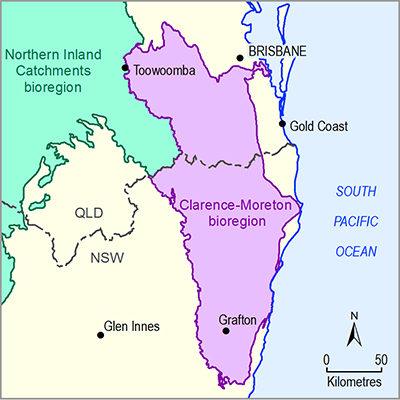# 2.6.2.8.1 Factors included in formal uncertainty analysis

Page 42 of 54

Section 2.6.2.7 described the available observations, predictions required of the model and sampling of parameter space as the design of experiment to train the emulators, as well as the sensitivity analysis based on these model runs. The same set of parameters as evaluated in the sensitivity analysis is considered in the formal uncertainty analysis, although the sensitivity analysis highlighted that a number of these parameters only have limited influence on the predictions.

As described in companion submethodology M09 (as listed in Table 1) for propagating uncertainty through models (Peeters et al., 2016) and in Section 2.6.2.1, the parameter space is constrained by the observations relevant to the predictions through a Markov chain Monte Carlo sampling using the Approximate Bayesian Computation (ABC) methodology (Beaumont et al., 2002; Vrugt and Sadegh, 2013). As in any Bayesian methodology, a set of prior parameter distributions needs to be defined that encapsulates the current information and knowledge, including correlation or covariance of parameters. This is described under Section 2.6.2.8.1.1 ‘Prior parameter distributions’.

These prior parameter distributions are constrained by observations with the ABC methodology to generate posterior parameter distributions. The posterior parameter distributions are then used to generate the final set of predictions. The process of constraining the prior parameter distributions by observations is described and discussed in Section 2.6.2.8.1.2 while the resulting posterior predictive distributions are detailed in Section 2.6.2.8.1.3

### 2.6.2.8.1.1 Prior parameter distributions

The parameterisation of the groundwater model, using multipliers of spatially variable properties and empirical relationships between depth and hydraulic conductivity, makes it very difficult to describe the distributions of the parameters directly from observations and measurements of the hydraulic properties. The Assessment team created the prior parameter distributions (blue lines in Figure 36) based on the available information in companion product 2.1-2.2 for the Clarence‑Moreton bioregion (Raiber et al., 2016a) and the initial model runs. The prior distributions are parameterised as a multivariate normal distribution with the mean of each parameter centred on the initial value listed in Table 8 in Section 2.6.2.7. The standard deviation is chosen such that more than 99% of the probability mass is within the range identified for the parameter. Some of the recharge parameters have an initial value very close to the lower boundary. For those the mean of the prior distribution is shifted to a higher value.

Hydraulic conductivity and storage are hydraulic properties that are both largely controlled by the lithological properties and cementation of the sediments or sedimentary rocks. It is, therefore, physically unlikely that high hydraulic conductivity values occur together with low storage values and vice versa (Delleur, 2007). For all layers, a covariance is specified between the hydraulic conductivity and storage parameters (Figure 37). The Pearson product moment correlation coefficient (ρ) is provided in the title of each subplot and is calculated as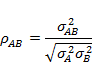(4)

with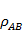the covariance between parameters A and B, and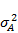and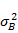the variance of parameters A and B, respectively. The covariance is purposefully chosen to result in a weak correlation between the variables. This allows a wide range of values to be chosen while reducing the likelihood of low and high values of hydraulic conductivity and storage occurring together.Figure 36 Histograms of prior (blue line) and posterior (green bars) parameter distributions of the groundwater model for the Clarence-Moreton bioregion

Posterior parameter distributions as obtained by the general objective function; Total sample size is 10,000; Refer to Table 4 in Section 2.6.2.4 for a detailed description and units of parameters

Data: Bioregional Assessment Programme (Dataset 1)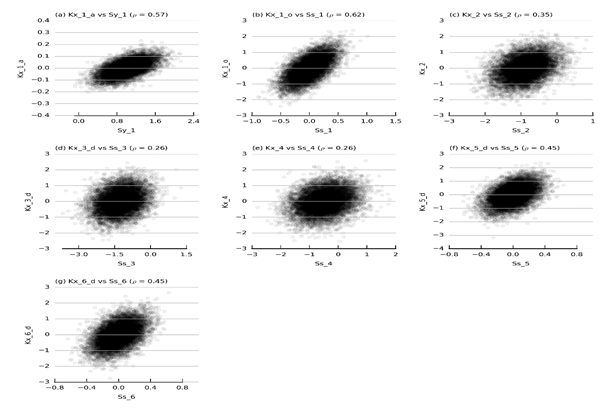Figure 37 Prior parameter covariance

Refer to Table 4 for a description of parameters; Ss = specific storage; Sy = specific yield; ρ is the Pearson product moment correlation coefficient

Data: Bioregional Assessment Programme (Dataset 1)

### 2.6.2.8.1.2 Posterior parameter distributions

The posterior parameter distributions are obtained through a Markov chain Monte Carlo sampling of the prior parameter distributions with a rejection sampler based on the ABC methodology. The rejection sampler only accepts a parameter combination, randomly drawn from the prior parameter distribution, if a model run with these parameters satisfies a predefined objective function threshold. This objective function is a summary of the model output that can be linked to groundwater level observations, the forecasted coal seam gas (CSG) water production rates and estimated baseflow rates. An example of an objective function is the mean absolute difference between observed and simulated groundwater levels. A crucial aspect in the ABC methodology is not only to define such an objective function, but to provide a threshold value as well that needs to be satisfied for a parameter set to be acceptable. Ideally this threshold is based on an independent estimate of the observation error.

For the Clarence‑Moreton bioregion, three types of observations are available to constrain the parameters for the predictions:

1. groundwater level observations (limited to layer 1 (alluvium, Lamington Volcanics and unconfined parts of the sedimentary bedrock))
2. an estimate of the surface water – groundwater flux
3. an estimate of the expected CSG water production rates.

Additionally, there are rules that can further constrain the parameter values based on the conceptual understanding and data analysis (see companion product 2.1-2.2 (Raiber et al., 2016a) and companion product 2.3 (Raiber et al., 2016b) for the Clarence‑Moreton bioregion), such as the condition that the hydraulic conductivity of layer 4 (Kangaroo Creek Sandstone) needs to be larger than the hydraulic conductivity of layer 3 (Bungawalbin Member).

The sensitivity analysis (Section 2.6.2.7.3) made it apparent that the various observation groups constrain different parameters, which are not necessarily the most important parameters for the predictions of change in groundwater level or surface water – groundwater flux. As a result, the Assessment team created an objective function tailored for each individual prediction. The simulated values at the locations and times in layer 1 where observations of groundwater level are available appeared to be mostly sensitive to recharge multipliers and drainage conductance of the drainage boundary at the top of the model. However, the predictions of change in groundwater level – especially in layers 2 to 6 (Grafton Formation to Walloon Coal Measures; see Section 2.6.2.3 for detailed layer structure) – are not sensitive to these parameters, and including the head observations in the objective function for these predictions will not constrain the relevant parameters. While this is mostly true for the predictions in layer 1 (alluvium, Lamington Volcanics and unconfined parts of the sedimentary bedrock), the sensitivity analysis indicates that the groundwater level predictions have some information to constrain the hydraulic properties of this layer, which are also important for the change in groundwater level predictions.

The objective function for predicted change in groundwater level due to the additional coal resource development in layers 2 to 6 and changes in surface water – groundwater flux consist of four acceptance criteria:

1. Hydraulic conductivity in layer 2 is larger than hydraulic conductivity in layer 3.
2. Hydraulic conductivity in layer 3 is less than hydraulic conductivity in layer 4.
3. The average difference between simulated and estimated CSG water production rates is less than 1000 m3/day. This ensures that the simulated CSG water production rate is within an order of magnitude of the estimated values.
4. The average difference between surface water – groundwater flux at the Casino surface water gauge for the months of September and October between 1983 and 2012 is less than 150 ML/day. During September and October rainfall is low and the contribution to streamflow from groundwater is highest (Rassam et al., 2014). Analysis of historical flow rates during periods of no rainfall at Casino indicated flow rates are rarely above 150 ML/day (Figure 21). This number can therefore be considered an upper bound to the surface water – groundwater flux.

Only parameter combinations that satisfy all four acceptance criteria are accepted in the posterior parameter distributions. Criteria 1 and 2 are implemented by directly rejecting any proposed sample from the prior parameter distributions that does not satisfy these criteria.

For criteria 3 and 4 the average difference between simulated and estimated CSG water production rates and the average difference between simulated and estimated surface water – groundwater flux at Casino is computed for all successful runs of the design of experiments. These values were used to create an emulator for criteria 3 and 4.

During the Monte Carlo simulation, a random sample of the prior parameter distribution is generated and evaluated with the two emulators. If all four acceptance criteria are satisfied, then the sample is accepted into the posterior parameter distribution. If not, then the sample is rejected. This process is repeated until a predefined number (in this case 10,000) of samples is accepted.

The green histograms in Figure 36 show the resulting posterior parameter distributions. As expected from the sensitivity analysis, the majority of parameters are unconstrained by the objective function and the posterior distribution for these is almost identical to the prior distributions. The parameters for which the posterior distribution is noticeably different to the prior distribution, are the drainage conductance of the CSG wells (DRN_C_1), the hydraulic conductivity and storage of layer 6 (Kx_6_d, Ss_6), the recharge multipliers of recharge zones 1 and 2 (RCH_1, RCH_2) and the riverbed conductance (rp1). For most of these parameters the change entails a shift of the mean of the distribution to a lower or higher value. For the recharge multipliers the mean does not change much, but the shape of the distribution changes with more values on the lower end of the range included in the parameter distributions.

For predictions of the change in groundwater level in layer 1 (alluvium, Lamington Volcanics and unconfined parts of the sedimentary bedrock), in addition to the four earlier defined criteria, the groundwater level observations are also used. The distribution of groundwater level observations in the model domain is not uniform, with a bias towards the alluvial system close to the rivers and a high density in the vicinity of Lismore. The groundwater level fluctuations observed close to a river are likely to be dominated by local processes, especially the dynamics of the river. Likewise, groundwater level fluctuations in the flat-lying areas in the east of the model domain are likely to be dominated by local recharge–discharge processes. These observations are not very likely to be of great value to constrain properties and processes relevant to groundwater levels in areas upstream with a more pronounced topography.

To acknowledge the difference in spatial support of each observation, an objective function is tailored for each prediction of groundwater level in layer 1 by weighting the difference between observed and simulated values of groundwater level based on the distance between the prediction location and the observation and the distance of the observation point to the nearest blue line network. The additional criterion for predictions of change in groundwater level in layer 1 is therefore defined as: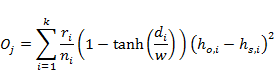(5)

where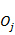is the criterion for prediction j,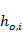is the ith observed groundwater level, and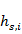is the simulated equivalent to this observation.is the distance (in km) of observationto the nearest blue line network whileis the distance (in km) between observationand prediction. Coefficient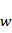controls the distance at which the weight of observationdrops to zero. To account for transient observations, the weights are divided by, the number of observations at the observation location. The tanh function allows the weight of an observation to decrease almost linearly with distance and to gradually become zero at a distance of approximately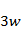. This is illustrated in Figure 38 for different values of.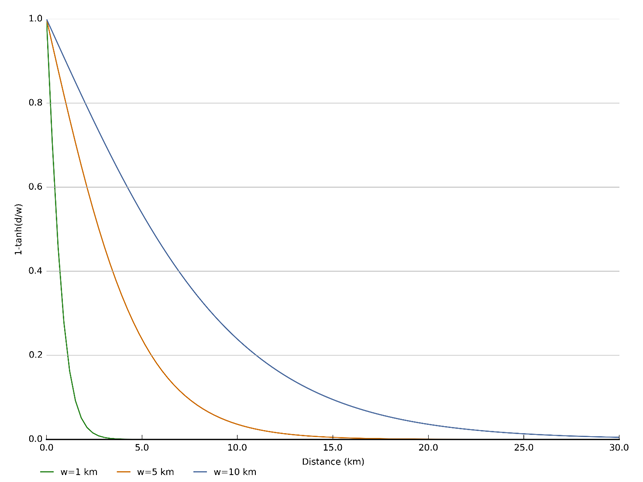Figure 38 Weights of observations in objective function in function of the distance between observation and prediction for different values of w

d = distance between observation and prediction (km); w = shortest distance between observation location and blue line network (km)

In the uncertainty analysis of the groundwater model for the Clarence‑Moreton bioregion the value ofis set equal to 5 km, which means that an observation has a weight of zero if the distance between the prediction and the observation is more than 15 km. The choice of this value is a pragmatic decision on behalf of the Assessment team. Ideally, the distance weighting function is chosen based on a spatial analysis of the available groundwater level observations. The sparsity of observations, especially transient observations, did not allow such an analysis. At a distance of 15 km the Assessment team judged that it is very likely that the groundwater system is affected by different stresses (recharge, surface water interaction) and has different hydraulic properties.

The threshold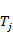, the upper limit, for each prediction is defined as:(6)

This means that any parameter combination that results in an average difference between observed and simulated groundwater level equal or less than 10 m is deemed acceptable. While this might be considered as a weak constraint, in a traditional calibration this would correspond to a normalised root mean squared error of 5% as the range of groundwater level observations spans about 180 m (Bioregional Assessment Programme, Dataset 1).Figure 39 (a) Groundwater level criterion threshold value for each prediction in layer 1 and (b) the fraction of successful design of experiment runs that meet the groundwater level acceptance criterion

Small threshold values in (a) indicate prediction locations that cannot be constrained by groundwater level observations. The acceptance rate in (b) is a proxy for conceptual model uncertainty: regions with very low acceptance rates indicate that it is not possible to locally match observations, despite the wide parameter range used. Regions with very high acceptance rates indicate that the model results agree with the observations, regardless of the parameter values.

Data: Bioregional Assessment Programme (Dataset 1)

Figure 39a shows the value of the threshold value for each individual prediction in layer 1, calculated with Equation 6. The value of the threshold as such is not that important, but high values indicate that a prediction is within the zone of influence of one or more observations. This means that the parameter distribution used for that prediction potentially can be constrained by groundwater level observations. Conversely, low values close to zero indicate that a prediction is far removed from any observation and therefore has a low potential to be constrained.

Figure 39b shows the fraction of successful runs of the design of experiment that meet the groundwater level acceptance criterion. High values, in excess of 0.8, indicate that a majority of the parameter combinations evaluated in the design of experiment result in groundwater level predictions that agree with the observations in that region (within the specified acceptable range). This means that the simulated groundwater levels in a region with high values of this acceptance rate are not very sensitive to the parameter values. This also means that the observations have limited potential to constrain the parameters in these regions and thus have limited potential to constrain the predictions. In other words, a good fit between modelled and simulated values in these areas does not guarantee reliable predictions.

In the groundwater model for the Clarence‑Moreton bioregion, the central region with high acceptance rates is probably due to a few observations that are close to a surface water boundary. The simulated groundwater levels in that region are mostly affected by the boundary conditions, rather than the parameter values.

Very low acceptance rates on the other hand, indicate that only a very limited number of parameter combinations result in simulated groundwater levels at the observation location that correspond to the observed values. As the parameter bounds in the design of experiment are deliberately chosen to be wide, low acceptance values indicate localised conceptual shortcomings of the model because it is not possible to get an acceptable correspondence between observed and simulated values. In some extreme cases, the acceptance rate is equal to zero, which occurs in three ellipse-shaped regions in the model. These are predictions within the overlapping zones of influence of two observation locations for which the simulated values cannot simultaneously agree with both observed groundwater levels. While this can be an indication of the shortcomings of the spatially uniform parameter multipliers within a layer, it is also likely that this is due to the boundary conditions, the local conceptualisation or even artefacts or errors in the observation database.

Figure 39b can be used as a proxy of the conceptual model uncertainty of the groundwater model. Very high acceptance rates indicate insensitivity to parameter values although the groundwater level predictions agree with observations. Low values, on the other hand, indicate regions of the model where it is unlikely to make groundwater level predictions that match the observations and where it is likely that the conceptualisation is locally inadequate. Three such areas can be identified in Figure 39b (black dots): north of the CSG development area, the western edge of the groundwater model and the eastern edge of the model closest to Byron Bay. While the extent and shape of these regions is determined by the weighting function, the presence of these zones indicates that for some sets of observations the model is not able to simultaneously match the observations within the prescribed error threshold.Figure 40 Convergence of the 95th percentile of the posterior distribution of additional drawdown with the number of samples from the posterior parameter distribution for model nodes pdm_324 (a) and pdm_1291 (b)

Additional drawdown is dmax, the maximum difference in drawdown between the coal resource development pathway and baseline, due to the additional coal resource development.

The posterior parameter distributions are sampled for each prediction until the 5th, 50th and 95th percentiles of the drawdown due to additional coal resource development (additional drawdown) stabilise. As the predictive distributions are heavily skewed to the right, that is, most of the predictions are close to zero with a small number of large drawdown values, the 5th and 50th percentile already stabilise for a small number of samples. The 95th percentile requires more samples to stabilise. This is illustrated in Figure 40 for the predictions of additional drawdown at model nodes pdm_324 and pdm_1291. The 95th percentile of dmax for pdm_324 already stabilises after 1500 samples, while at least 6000 samples are required for the prediction at pdm_1291 to stabilise. Do note that for both model nodes the 95th percentile of dmax is within 5 cm of the final stabilised value after 500 samples from the posterior parameter distribution.

### 2.6.2.8.1.3 Predictions

For 1462 locations within the model domain (see Figure 42), predictions of additional drawdown and year of maximum change are made by sampling the appropriate posterior parameter distribution. Figure 41 shows histograms of the 5th, 50th and 95th percentile of drawdown summarised per model layer. Figure 41e shows that in layer 3 the 5th percentile and the median predicted drawdowns (50th percentile) are less than 5 cm at all 29 model nodes. At 10 model nodes the 95th percentile of drawdown is also less than 5 cm. For 13 model nodes the 95th percentile of dmax is between 5 and 10 cm, for 4 model nodes between 10 and 15 cm, and for 2 model nodes the 95th percentile of dmax is between 15 and 20 cm.

The 5th percentile of additional drawdown in all layers at the model nodes is less than 5 cm. The median dmax is mostly less than 5 cm in all layers, with the exception of layers 1 and 2, where there are about 30 model nodes in each layer for which the median dmax is between 5 and 15 cm. The 95th percentile of additional drawdown at the model nodes can reach values up to 1 m in layers 1 and 2. In the Walloon Coal Measures there is one model node for which the 95th percentile of dmax is equal to 2.2 m. These plots highlight that the posterior distributions of predicted additional drawdown are very skewed, with most of the simulations resulting in dmax close to 0 m, with a smaller proportion of drawdowns up to 1 m in model nodes in layer 1 and 2, up to 20 cm in model nodes in layer 3 and 4 and up to 2.2 m in model nodes in layer 6.

The posterior distributions for year of maximum change (tmax) are less skewed (Figure 41). The earliest year of maximum change shown in Figure 41 is 2038, which corresponds to the end time of the planned CSG exploitation. For most of the models nodes, however, the year of maximum change is at or beyond 2102. A considerable number of model nodes in layers 1 to 4 have median predicted year of maximum change values in the decades following cessation of active depressurisation, while the median values in layer 6 are all shown as 2102. Several model nodes in layers 1 to 4 are within or close to the proposed CSG development area. The median tmax values less than 2102 illustrate the vertical propagation of the cone of depression through the sedimentary basin.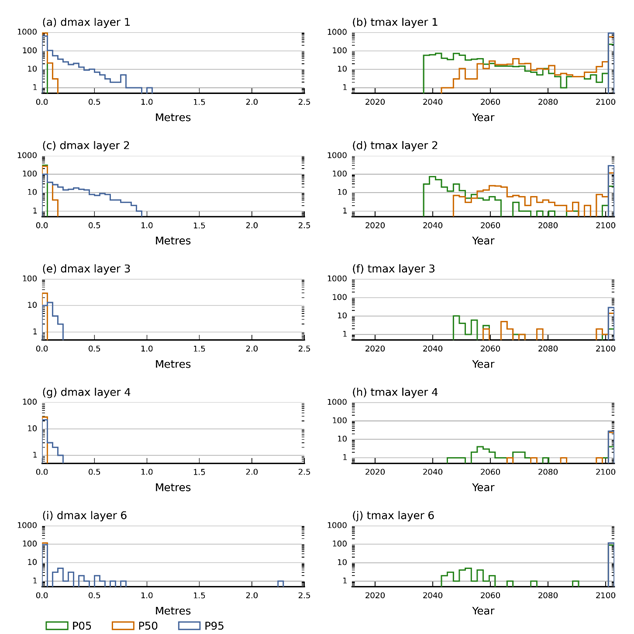Figure 41 Histograms of 5th, 50th and 95th percentile of additional drawdown (left) and year of maximum change (right)

Additional drawdown is dmax, the maximum difference in drawdown between the coal resource development pathway and baseline, due to the additional coal resource development; tmax = year of maximum change; 5th percentile of dmax is not visible as it is covered by the line for the 50th percentile

Data: Bioregional Assessment Programme (Dataset 1)Figure 42 Probability (P) of exceeding 0.2 m drawdown at the model nodes in layer 2 (a), layer 3 (b), layer 4 (c) and layer 6 (d)

Additional drawdown is dmax, the maximum difference in drawdown between the coal resource development pathway and baseline, due to the additional coal resource development (ACRD).

Data: Bioregional Assessment Programme (Dataset 1)

Figure 42 shows the probability of drawdown exceeding 0.2 m for each layer. This threshold corresponds to the impact threshold in the NSW Aquifer Interference Policy (NSW DPI, 2012) for impact on groundwater-dependent ecosystems. Figure 43 shows the gridded probability of additional drawdown exceeding 20 cm for layer 1. As such, this threshold is only relevant for layer 1, as the predictions in layers 2 to 6 are all at licensed groundwater bores, where the threshold for impact is 2 m. As shown in Figure 41, the 95th percentile of additional drawdown only exceeds the 2 m threshold at a single model node in layer 6. The exceedance probabilities of 20 cm drawdown show that model nodes close to the proposed CSG area have the highest probabilities of exceeding 20 cm additional drawdown. The highest probability of exceeding 20 cm drawdown is recorded in layer 2 and is 38%. The predicted additional drawdown in the model nodes in layer 6 are mostly small as the majority of model nodes are situated more than 10 km from the centre of the proposed CSG development area.

Figure 43 shows that the area where the probability of exceeding 20 cm additional drawdown exceeds 0.05 extends at most 10 km west of the CSG development area. The map also shows the mitigating effect of the major rivers on additional drawdown.Figure 43 Probability of exceeding 0.2 m additional drawdown in layer 1

Additional drawdown is dmax, the maximum difference in drawdown between the coal resource development pathway and baseline, due to the additional coal resource development (ACRD).

These results are generally comparable with the findings of the groundwater modelling carried out for the Metgasco IES (Parsons Brinckerhoff, 2013). The BA modelling indicates a non-negligible probability of exceeding 20 cm drawdown beyond the additional coal resource development area (Figure 42a), especially to the west. The Metgasco model shows very limited impact outside the additional coal resource development area in the watertable aquifer. The Metgasco model predicts that the drawdown due to the CSG abstraction is generally less than 0.2 m in layer 1 and the Piora Member (layer 2). The 95th percentile of additional drawdown predicted by the bioregional assessment (BA) model at any model nodes in layer 1 or layer 2 is not in excess of 0.48 m. While there are no model nodes in the Walloon Coal Measures within the additional coal resource development area, the relatively small changes in the Walloon Coal Measures at the existing model nodes agree with the rapid decline of drawdown due to the low hydraulic conductivity in the Walloon Coal Measures simulated in Parsons Brinckerhoff (2013).

Last updated:
7 January 2019